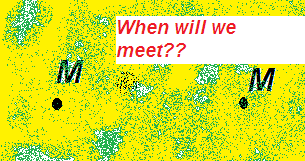# The time before they met1) Two bodies of mass M are separated by a distance d. Consider only the gravitational force between bodies, find the time for them to meet. if the answer is $t=x\sqrt{\frac{d^{3}}{GM}}$ find last digit of [10000x] The value of pi should not be approximated for the answer

×